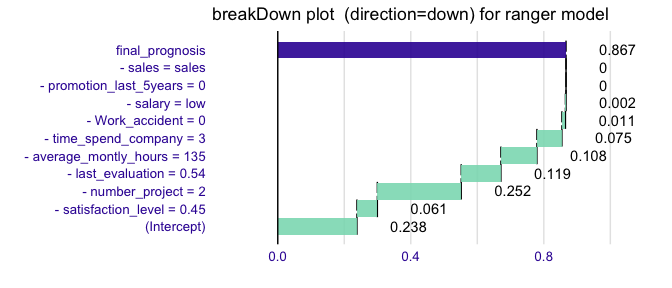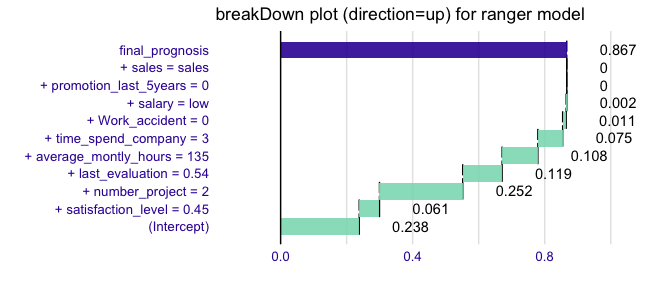# model agnostic breakDown plots for ranger

#### 2020-04-04

Here we will use the HR churn data (https://www.kaggle.com/) to present the breakDown package for ranger models.

The data is in the breakDown package

library(breakDown)
#>   satisfaction_level last_evaluation number_project average_montly_hours
#> 1               0.38            0.53              2                  157
#> 2               0.80            0.86              5                  262
#> 3               0.11            0.88              7                  272
#>   time_spend_company Work_accident left promotion_last_5years sales salary
#> 1                  3             0    1                     0 sales    low
#> 2                  6             0    1                     0 sales medium
#> 3                  4             0    1                     0 sales medium

Now let’s create a ranger classification forest for churn, the left variable.

library(ranger)
#>
#> Attaching package: 'ranger'
#> The following object is masked from 'package:randomForest':
#>
#>     importance
HR_data$left <- factor(HR_data$left)
model <- ranger(left ~ ., data = HR_data, importance = 'impurity', probability=TRUE, min.node.size = 2000)

predict.function <- function(model, new_observation) predict(model, new_observation, type = "response")\$predictions[,2]

predict.function(model, HR_data[11,])
#>         1
#> 0.8667675

But how to understand which factors drive predictions for a single observation?

With the breakDown package!

Explanations for the trees votings.

library(ggplot2)

explain_1 <- broken(model, HR_data[11,-7], data = HR_data[,-7],
predict.function = predict.function,
direction = "down")
explain_1
#>                              contribution
#> (Intercept)                         0.238
#> - satisfaction_level = 0.45         0.061
#> - number_project = 2                0.252
#> - last_evaluation = 0.54            0.119
#> - average_montly_hours = 135        0.108
#> - time_spend_company = 3            0.075
#> - Work_accident = 0                 0.011
#> - salary = low                      0.002
#> - promotion_last_5years = 0         0.000
#> - sales = sales                     0.000
#> final_prognosis                     0.867
#> baseline:  0
plot(explain_1) + ggtitle("breakDown plot  (direction=down) for ranger model")explain_2 <- broken(model, HR_data[11,-7], data = HR_data[,-7],
predict.function = predict.function,
direction = "up")
explain_2
#>                              contribution
#> (Intercept)                         0.238
#> + satisfaction_level = 0.45         0.061
#> + number_project = 2                0.252
#> + last_evaluation = 0.54            0.119
#> + average_montly_hours = 135        0.108
#> + time_spend_company = 3            0.075
#> + Work_accident = 0                 0.011
#> + salary = low                      0.002
#> + promotion_last_5years = 0         0.000
#> + sales = sales                     0.000
#> final_prognosis                     0.867
#> baseline:  0
plot(explain_2) + ggtitle("breakDown plot (direction=up) for ranger model")